Family and age ratios

Age of father and son are in the ratio 10:3; age of father and daughter are in the ratio 5:2. How old is father and son, if daughter is 20 years old? In what ratio is age sister and brother?

Result

d =  20
o =  50
s =  15
x =  4:3

Solution:

3o = 10s
2o = 5d
d = 20

3o-10s = 0
5d-2o = 0
d = 20

d = 20
o = 50
s = 15

Calculated by our linear equations calculator.Leave us a comment of this math problem and its solution (i.e. if it is still somewhat unclear...):Be the first to comment!To solve this verbal math problem are needed these knowledge from mathematics:

Do you have a linear equation or system of equations and looking for its solution? Or do you have quadratic equation?

Next similar math problems:

1. Family 8Father is 38 years old, daughter 12, son 14. How many years will father have as many years as his children together?
2. Family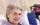Mom is 39 years old. Karl is eight years and Paul 4 years. For many years mother would old just like two children together?
3. Brothers and sistersLenka has as many brothers as sisters. Her brothers have twice as many sisters as brothers. How many brothers and sisters are in the family?
4. The classroom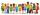In the seventh class the number of boys and girls are at a ratio of 4:5. Boys are 16. How many total students are in the classroom?
5. Two ages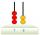Gabrielle is 9 years younger than Mikhail. The sum of their ages is 87. What is Mikhail's age?
6. SuzanSusan's age will be after 12 years four times as much as twelve years ago. How old is Susan now?
7. Negative in equation2x + 3 + 7x = – 24, what is the value of x?
8. Tickets 3A total of 645 tickets were sold for the school play. They were either adult tickets or student tickets. There were 55 fewer student tickets sold than adult tickets. How many adult tickets were sold?
9. The store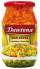The store received the same number of cans of peas and corn. The first day sold 10 cans of peas and 166 cans ofcorn so that left 5 times more peas than corn cans. How many cans of each kind were in the store?
10. Simple equationSolve the following simple equation: 2. (4x + 3) = 2-5. (1-x)
11. Box of chocolatesIn a box of chocolates was 16 candies. Christopher and Luke they were distributed: a) Christopher had about 4 candies over Luke, b) Christopher had about 6 candies less than Luke, c) Christopher had 3 times more sweet than Luke. How many had each boy can
12. Simple equationSolve for x: 3(x + 2) = x - 18
13. Forest nurseryIn the forest nursery after winter, they found that 1/10 stems died out of them. For them, they land 193 new spruces. How many spruces are in the forest nursery?
14. Chicken and rabbits (classic)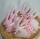Grandmother breeding chickens and rabbits. It found that the yard has 35 heads and 108 legs. How many chickens and rabbits breeding in the yard?
15. Every day 7 pagesAdelka reads the book every day 7 pages. When she reads one more page a day she will read it three days earlier. How long will Adelka read a book? How much does a book of pages have?
16. Football seasonThroughout the football season gave Adam the 23 goals more than Brano and 16 goals less than Karol. Edo gave the 6 goals more Dušan. Karol gave 5 more than Edo. Brano and Edo gave together 17 goals. How many goals gave each boy and how many goals gave the.
17. EquationSolve the equation: 1/2-2/8 = 1/10; Write the result as a decimal number.